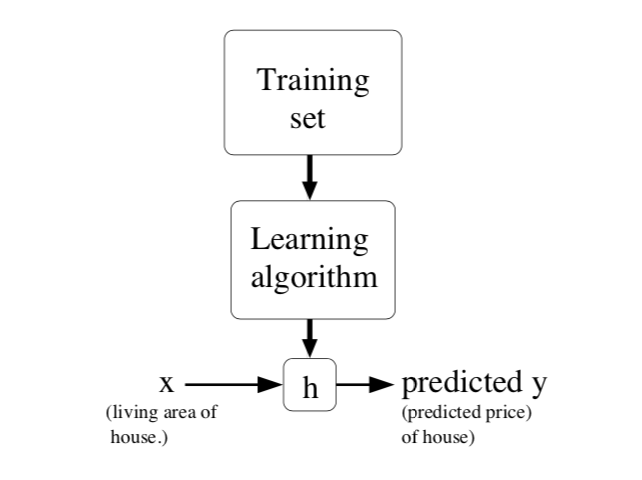# 监督学习

$${(x^{(i)}, y^{(i)}); i = 1,…,m}$$# 线性回归

$$h_\theta(x) = \theta_0 + \theta_1x_1 + \theta_2x_2$$

$$h(x) = \sum_{i=0}^{n}\theta_ix_i = \theta^Tx$$

$$J(\theta) = \frac{1}{2}\sum_{i=1}^{m}(h_\theta(x^{(i)}) - y^{(i)})^2$$

## LMS算法

$$\theta_j := \theta_j - \alpha\frac{\partial}{\partial \theta_j}J(\theta)$$

\begin{align}
\frac{\partial}{\partial \theta_j}J(\theta)
&= \frac{\partial}{\partial \theta_j} \frac{1}{2} (h_\theta(x) - y)^2 \\
&= 2 \frac{1}{2} (h_\theta(x) - y) \frac{\partial}{\partial \theta_j} (h_\theta(x) - y) \\
&= (h_\theta(x) - y) \frac{\partial}{\partial \theta_j} (\sum_{i=0}^{n} \theta_i x_i - y) \\
&= (h_\theta(x) - y)x_j
\end{align}

$$\theta_j := \theta_j + \alpha (y^{(i)} - h_\theta(x^{(i)})) x_j^{(i)}$$

$$\theta_j := \theta_j + \alpha \sum_{i=1}^{m}(y^{(i)} - h_\theta(x^{(i)})) x_j^{(i)}$$

## 概率解释

$$y^{(i)} = \theta^T x^{(i)} + \epsilon^{(i)}$$

$$p(\epsilon^{(i)}) = \frac{1}{\sqrt{2\pi}\sigma}exp(-\frac{(\epsilon^{(i)})^2}{2\sigma^2})$$

$$p(y^{(i)}|x^{(i)};\theta) = \frac{1}{\sqrt{2\pi}\sigma} exp(- \frac{(y^{(i)} - \theta^Tx^{(i)})^2}{2\sigma^2})$$

$$L(\theta) = L(\theta; X, \vec{y}) = p(\vec{y}|X; \theta)$$

\begin{align} L(\theta) &= \prod_{i=1}^{m}p(y^{(i)} | x^{(i)}; \theta) \\ &= \prod_{i=1}^{m} \frac{1}{\sqrt{2\pi}\sigma} exp(- \frac{(y^{(i)} - \theta^Tx^{(i)})^2}{2\sigma^2}) \end{align}

\begin{align} l(\theta) &= logL(\theta) \\ &= log\prod_{i=1}^{m} \frac{1}{\sqrt{2\pi}\sigma} exp(- \frac{(y^{(i)} - \theta^Tx^{(i)})^2}{2\sigma^2}) \\ &= \sum_{i=1}^{m}log \frac{1}{\sqrt{2\pi}\sigma} exp(- \frac{(y^{(i)} - \theta^Tx^{(i)})^2}{2\sigma^2}) \\ &= m \cdot log \frac{1}{\sqrt{2\pi}\sigma} - \frac{1}{\sigma^2} \cdot \frac{1}{2} \sum_{i=1}^{m} (y^{(i)} - \theta^Tx^{(i)})^2 \end{align}

$$\frac{1}{2} \sum_{i=1}^{m} (y^{(i)} - \theta^Tx^{(i)})^2$$

## 局部加权线性回归

LWR(Locally weighted linear regression)算法中，目标是使如下式子最小化

$$\sum w^{(i)}(y^{(i)} - \theta^Tx^{(i)})^2$$

$$w^{(i)} = exp(- \frac{(x^{(i)} - x)^2}{2\tau ^2})$$

LWR是非参数化学习算法。所谓参数化(parametric)学习算法，即训练出算法的参数后，可以使用算法对新的输入进行结果预测，不需要保留训练集，比如原始的线性回归算法。非参数化(non-parametric)学习算法刚好相反，需始终保留训练集

0%